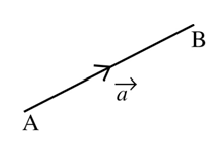# Introduction To Vectors

Physical quantities are divided into two categories: Scalar quantities and Vector quantities.

### Scalar

A quantity having only magnitude is called a scalar. It is not related to any fixed direction in space.

Examples: mass, volume, density, work, temperature, distance, area, real numbers

To represent a scalar quantity, you assign a real number to it, which gives its magnitude in terms of a certain basic unit of a quantity.

### Vector

A quantity having both magnitude and direction is called a vector.

Examples: displacement, velocity, acceleration, momentum, force, moment of a force, weight

Vectors are represented by directed line segments such that the length of the line segment is the magnitude of the vector and the direction of arrow marked at one end denotes the direction of the vector.### Equality of Vectors

Two vectors are equal, if they have same magnitude and same direction.